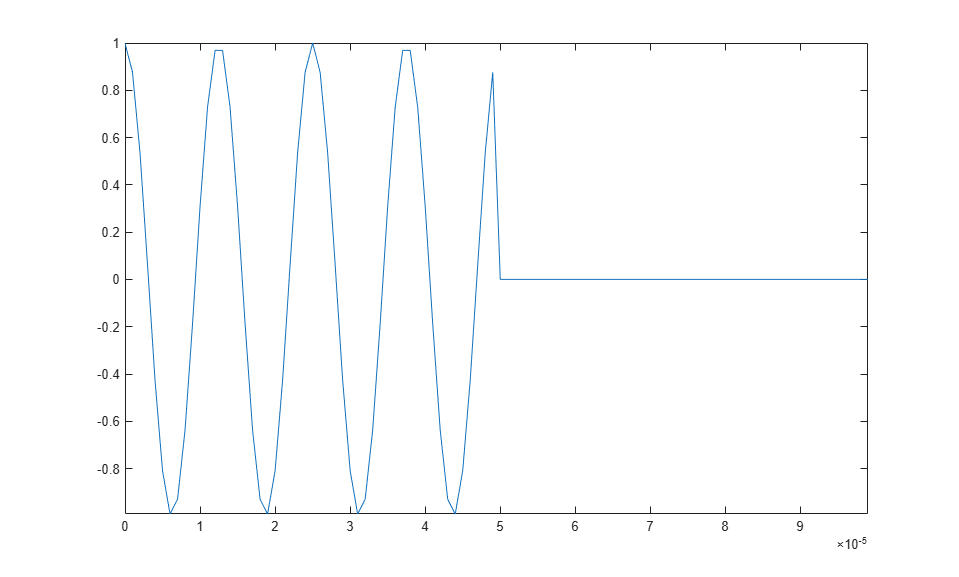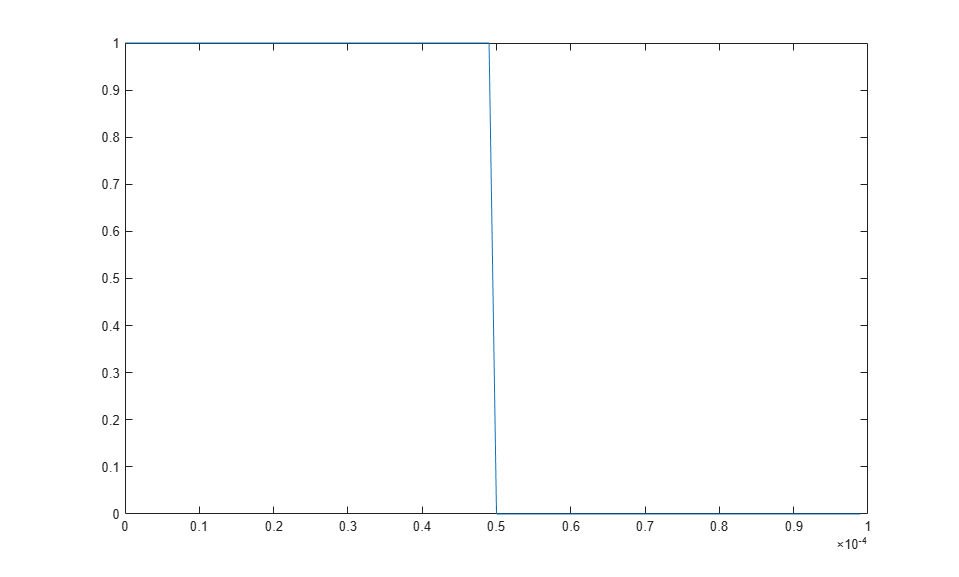## Stepped FM Pulse Waveforms

A stepped frequency pulse waveform consists of a series of N narrowband pulses. The frequency is increased from step to step by a fixed amount, Δf, in Hz.

Similar to linear FM pulse waveforms, stepped frequency waveforms are a popular pulse compression technique. Using this approach enables you to increase the range resolution of the radar without sacrificing target detection capability.

To create a stepped FM pulse waveform, use `phased.SteppedFMWaveform`.

The stepped frequency pulse waveform has the following modifiable properties:

• `SampleRate` — Sampling rate in Hz

• `PulseWidth` — Pulse duration in seconds

• `PRF` — Pulse repetition frequency in Hz

• `FrequencyStep` — Frequency step in Hz

• `NumSteps` — Number of frequency steps

• `OutputFormat` — Output format in pulses or samples

• `NumSamples` — Number of samples in the output when the `OutputFormat` property is `'Samples'`

• `NumPulses` — Number of pulses in the output when the `OutputFormat` property is `'Pulses'`

### Create and Plot Stepped FM Pulse Waveform

This example shows how to create and plot a 5-step stepped FM pulse waveform using the `phased.SteppedFM` System object™. Set the pulse width (duration) to 50 µs, the pulse repetition frequency (PRF) to 10 kHz, and the frequency step size to 20 kHz. The sampling rate is 1 MHz. By default, the `OutputFormat` property is set to `'Pulses'` and `NumPulses` is one.

```waveform = phased.SteppedFMWaveform('SampleRate',1e6,... 'PulseWidth',50e-6,'PRF',10e3,... 'FrequencyStep',20e3,'NumSteps',5); ```

Use the `bandwidth` method to show that the bandwidth of the stepped FM pulse waveform equals the product of the frequency step size and the number of steps.

```bandwidth(waveform) ```
```ans = 100000 ```

Because the `OutputFormat` property is set to 'Pulses' and the `NumPulses` property is set to one, executing the System object returns one pulse repetition interval (PRI). The pulse duration within that interval is set by the `PulseWidth` property. The signal in the remainder of the PRI consists of zeros.

The frequency of the initial pulse is zero Hz (DC). Each time you execute the System object, the frequency of the narrowband pulse increments by the value of the `FrequencyStep` property. If you execute the System object more times than the value of the `NumSteps` property, the process repeats, starting over with the DC pulse.

Execute the System object to return successively higher frequency pulses. Plot the pulses one by one in the same figure window. Pause the loop to visualize the increment in frequency with each execution of the System object. Execute the System object one more time than the number of pulses to demonstrate that the process starts over with the DC pulse.

This figure shows the pulse plot for the last iteration of the loop.

```t = unigrid(0,1/waveform.SampleRate,1/waveform.PRF,'[)'); for i = 1:waveform.NumSteps plot(t,real(waveform())) pause(0.5) axis tight end ```This plot shows how the pulse returns to its DC value.

```plot(t,waveform()) ```